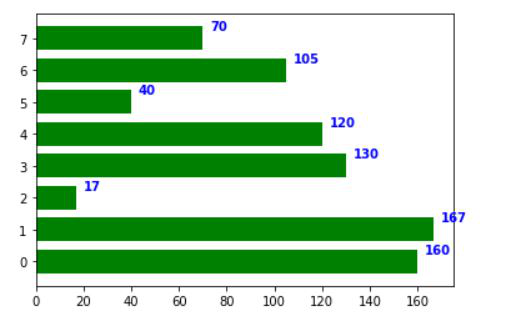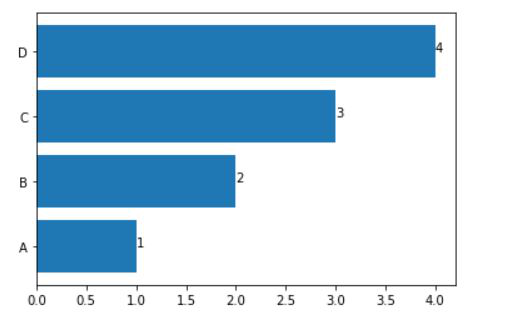Open In App
Related Articles
• Write an Interview Experience

# How to display the value of each bar in a bar chart using Matplotlib?

In this article, we are going to see how to display the value of each bar in a bar chart using Matplotlib. There are two different ways to display the values of each bar in a bar chart in matplotlib –

Example 1: Using matplotlib.axes.Axes.text() function:

This function is basically used to add some text to the location in the chart. This function return string, this is always used with the syntax “for index, value in enumerate(iterable)” with iterable as the list of bar values to access each index, value pair in iterable so at it can add the text at each bar.

## Python3

 `import` `os``import` `numpy as np``import` `matplotlib.pyplot as plt` `x ``=` `[``0``, ``1``, ``2``, ``3``, ``4``, ``5``, ``6``, ``7``]``y ``=` `[``160``, ``167``, ``17``, ``130``, ``120``, ``40``, ``105``, ``70``]``fig, ax ``=` `plt.subplots()``width ``=` `0.75``ind ``=` `np.arange(``len``(y))` `ax.barh(ind, y, width, color ``=` `"green"``)` `for` `i, v ``in` `enumerate``(y):``    ``ax.text(v ``+` `3``, i ``+` `.``25``, ``str``(v),``            ``color ``=` `'blue'``, fontweight ``=` `'bold'``)``plt.show()`

Output:Example 2: Use matplotlib.pyplot.text() function:

Call matplotlib.pyplot.barh(x, height) with x as a list of bar names and height as a list of bar values to create a bar chart. Use the syntax “for index, value in enumerate(iterable)” with iterable as the list of bar values to access each index, value pair in iterable. At each iteration, call matplotlib.pyplot.text(x, y, s) with x as value, y as index, and s as str(value) to label each bar with its size.

## Python3

 `import` `matplotlib.pyplot as plt``x ``=` `[``"A"``, ``"B"``, ``"C"``, ``"D"``]``y ``=` `[``1``, ``2``, ``3``, ``4``]``plt.barh(x, y)` `for` `index, value ``in` `enumerate``(y):``    ``plt.text(value, index,``             ``str``(value))` `plt.show()`

Output: